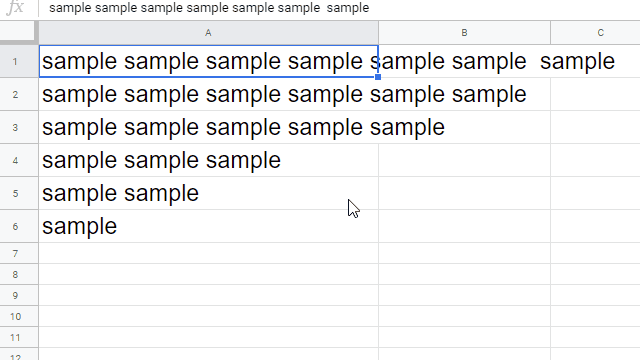Gists

This is a sample script for adjusting the text length to fit in the cell width on Google Spreadsheet using Google Apps Script. In this case, in order to fit to the cell width, the font size is changed.

## Issue and workaround:

Unfortunately, in the current stage, there are no methods for automatically resize the font size for fitting in the cell width in the Spreadsheet service. So in this case, it is required to think of the workaround. But the direction for calculating the length of texts in the unit of pixel cannot be directly used. Because as a test case, when I compared the text length (pixel) calculated from the font size and the cell width (pixel), those were different. By this, here, I would like to introduce a workaround using other direction. The base flow of this workaround is as follows.

1. Retrieve the cell width.
2. Automatically resizing the cell width using autoResizeColumn.
3. Calculate the ratio of the original width and the resized width of the cell.
4. Change the font size using the ratio.

By this flow, the text length to fit in the cell width can be automatically adjusted.

## Sample script:

function myFunction() {
const autoResizeFont = (range, toLarge) => {
const sheet = range.getSheet();
const ss = sheet.getParent();
const startColumn = range.getColumn();
const endColumn = range.getColumn() + range.getNumColumns();
const startRow = range.getRow();
const endRow = range.getRow() + range.getNumRows();
const columnObj = [];
for (let c = startColumn; c < endColumn; c++) {
columnObj.push({
column: c,
width: sheet.getColumnWidth(c),
});
}
const tempSheet = ss.insertSheet("tempForAutoresizeFont");
sheet.activate();
const tempRange = tempSheet.getRange("A1");
for (let r = startRow; r < endRow; r++) {
for (let c = 0; c < columnObj.length; c++) {
const srcRange = sheet.getRange(r, columnObj[c].column);
tempSheet.setColumnWidth(1, columnObj[c].width);
srcRange.copyTo(tempRange);
tempSheet.autoResizeColumn(1);
const resizedWidth = tempSheet.getColumnWidth(1);
tempSheet.setColumnWidth(1, columnObj[c].width);
const ratio = columnObj[c].width / resizedWidth;
if (ratio > 1 && !toLarge) continue;
const fontSize = srcRange.getFontSize();
srcRange.setFontSize(Math.ceil(fontSize * ratio));
}
}
ss.deleteSheet(tempSheet);
};

const sheet = ss.getSheetByName("Sheet1");
const targetRange = "A1:A6";
const toLarge = true;
autoResizeFont(sheet.getRange(targetRange), toLarge);
}

• Please set targetRange.
• In this sample script, a temporal sheet is created and the ratio of the original width and the resized width of the cell is calculated using the temporal sheet.
• When toLarge is true, when the text length is smaller than the cell width, the font size of the text becomes large. By this, the text length is matched to the cell width. When toLarge is false, when the text length is smaller than the cell width, the font size of the text not changed.

## Result:

In this demonstration, the lengths of texts in the cells “A1:A6” are matched to the cell width by changing the font size. In this case, toLarge is true.## Note:

• In this case, the font size for setFontSize(size) is “Integer”. By this, the text length might not be exactly the same because the font size is required to be the integer type. So please be careful this.

## For Library

This workaround was also implemented to “RichTextApp” of the Google Apps Script library.